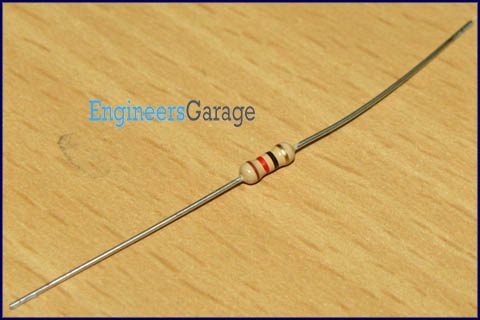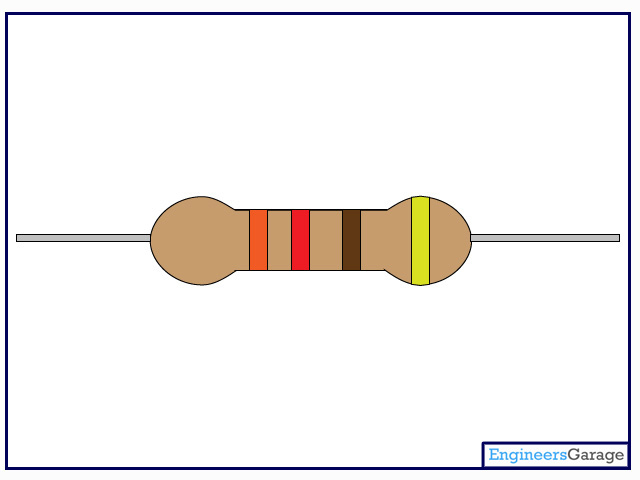﻿ Resistor

# Resistor

Written By:

• Kushagra

### Image:Resistor is a passive component used to control current in a circuit. Its resistance is given by the ratio of voltage applied across its terminals to the current passing through it. Thus a particular value of resistor, for fixed voltage, limits the current through it. They are omnipresent in electronic circuits.

The different value of resistances are used to limit the currents or get the desired voltage drop according to the current-voltage rating of the device to be connected in the circuit. For example, if an LED of rating 2.3V and 6mA is to be connected with a supply of 5V, a voltage drop of 2.7V (5V-2.3V) and limiting current of 6mA is required. This can be achieved by providing a resistor of 450connected in series with the LED.

Resistors can be either fixed or variable. The low power resistors are comparatively smaller in size than high power resistors. The resistance of a resistor can be estimated by their colour codes or can be measured by a multimeter. There are some non linear resistors also whose resistance changes with temperature or light. Negative temperature coefficient (NTC), positive temperature coefficient (PTC) and light dependent resistor (LDR) are some such resistors. These special resistors are commonly used as sensors. Read and learn about internal structure and working of a resistor.

### Pin Diagram: